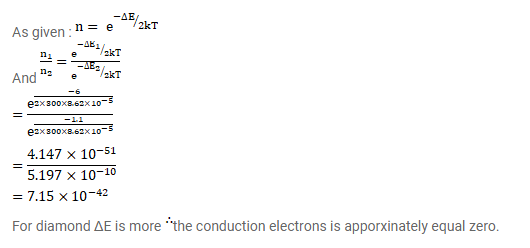# Let ΔE denote the energy gap between the valence band and the conduction band.

Question:

Let $\Delta \mathrm{E}$ denote the energy gap between the valence band and the conduction band. The population of conduction electrons (and of the holes) is roughly proportional to $\mathrm{e}^{-\Delta \mathrm{E} / 2 \mathrm{kT}}$. Find the ratio of the concentration of conduction electrons in diamond to that in silicon at room temperature $300 \mathrm{~K} . \Delta \mathrm{E}$ for silicon is $1.1 \mathrm{eV}$ and for diamond is $6.0 \mathrm{eV}$. How many conduction electrons are likely to be in one cubic metre of diamond?

Solution: